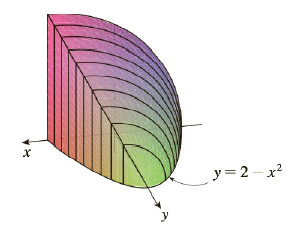Chapter 5.2, Problem 60E

Chapter
Section
Textbook Problem

# Find the volume of the described solid S.The base of S is the region enclosed by y = 2 − x 2 and the x-axis. Cross-sections perpendicular to the y-axis are quarter-circles.To determine

To find:

The volume of solid S whose base is the region enclosed by y=2-x2 and x-axis  and cross sections are perpendicular to the y-axis are quarter circles.

Explanation

1) Concept:

Definition of Volume:

Let S be a solid that lies between x=a and  x=b. If the cross sectional area of S in the plane Px, through x and perpendicular to the x-axis, is  A(x), where A is continuous function, then the volume of S is

V=limni=1nAxi*x=abAxdx

2) Calculations:

The cross sections are perpendicular to the y-axis.

Therefore, solve the equation of parabola for x.

y=2-x2

x= ±2-y

So the perpendicular distance of a point from y-axis is 2-y. So, to get length of base of cross section double the distance from above.

Thus cross section of solid S at y is a quarter ciecle with radius 22-y.

Area of circle is πr2.

Therefore, area of a quarter circle is 14πr2

### Still sussing out bartleby?

Check out a sample textbook solution.

See a sample solution

#### The Solution to Your Study Problems

Bartleby provides explanations to thousands of textbook problems written by our experts, many with advanced degrees!

Get Started

#### In Exercises 5-8, graph the given function or equation. 2x3y=12

Finite Mathematics and Applied Calculus (MindTap Course List)

#### Let f be the function defined by f(x)={x2+1ifx0xifx0 Find f(2), f(0), and f(1).

Applied Calculus for the Managerial, Life, and Social Sciences: A Brief Approach

#### Given the Taylor Series , a Taylor series for ex/2 is:

Study Guide for Stewart's Single Variable Calculus: Early Transcendentals, 8th

#### The quadratic approximation for at a = 1 is:

Study Guide for Stewart's Multivariable Calculus, 8th